# CBSE Class 12 Physics Chapter 5 Revision Notes

Chapter 5: Magnetism and Matter

• M = m x 2I is the magnetic dipole moment of a magnetic dipole, where m is the pole strength and 2I is the dipole length directed from S to N.
• A-m2 or J/T is the SI unit for magnetic dipole moment.
• It is a vector quantity that runs from the South Pole to the North Pole.

### Coulomb’s Law in Magnetism

• The magnitude of force acting between two magnetic poles is given by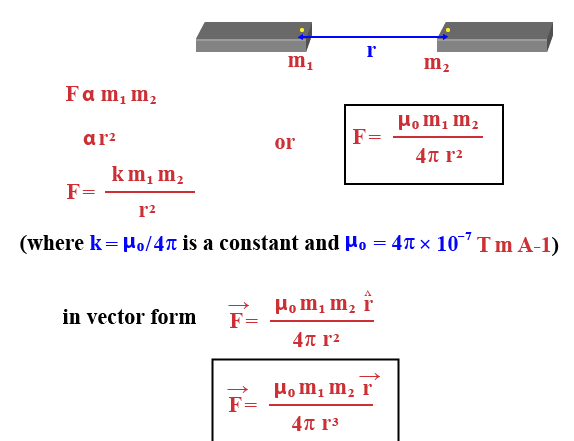Source

### Magnetic Field Lines

• These are imaginary lines that depict the magnetic field inside and around the magnet in a pictorial manner.

The following are their characteristics:

(i) These lines form closed loops that are continuous.

(ii) The tangent to the field line determines the field’s direction at that point.

(iii) The magnetic field will be stronger as the density of the lines increases.

(iv) These lines do not cross each other.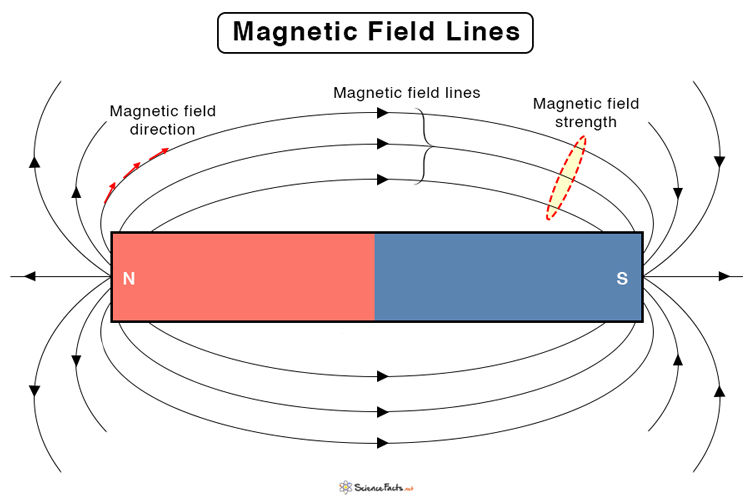Source

• The magnetic field between the poles of the dipole is in the opposite direction of the magnetic moment (South to North), whereas it is in the same direction as the magnetic moment inside the current loop.
• A magnetic dipole’s potential energy in a magnetic field is given by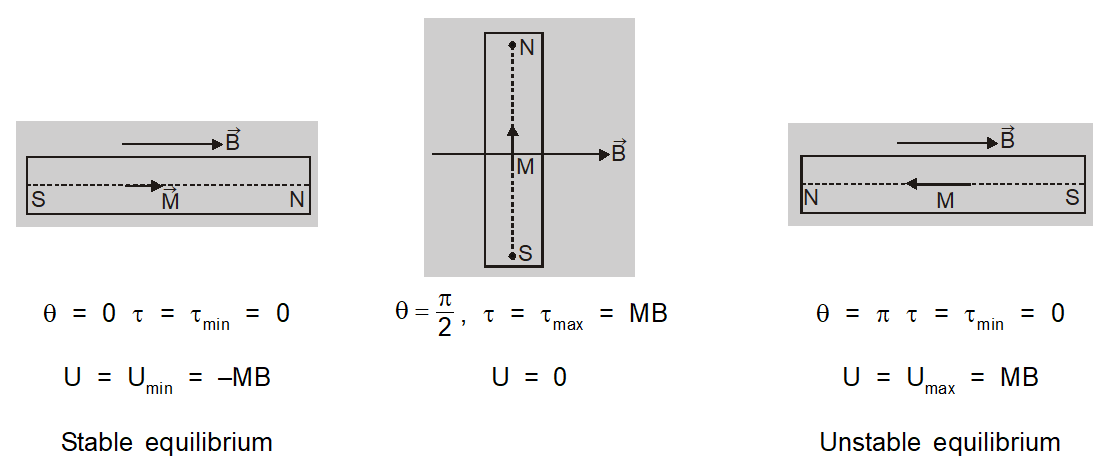Source

• The current loop behaves like a magnetic dipole, with M=IA as the dipole moment.
• The right hand thumb rule can be used to determine the direction of the dipole 11.
• Freely Suspended Magnet Oscillation (SHM) describes the oscillations of a free-floating magnet (magnetic dipole) in a uniform magnetic field.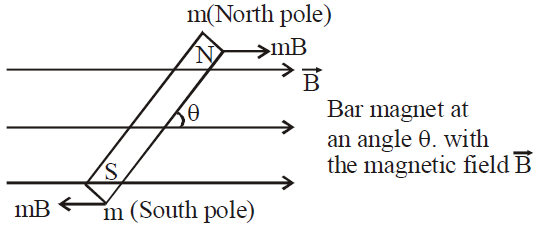Source

• Equivalent Solenoid with a Bar Magnet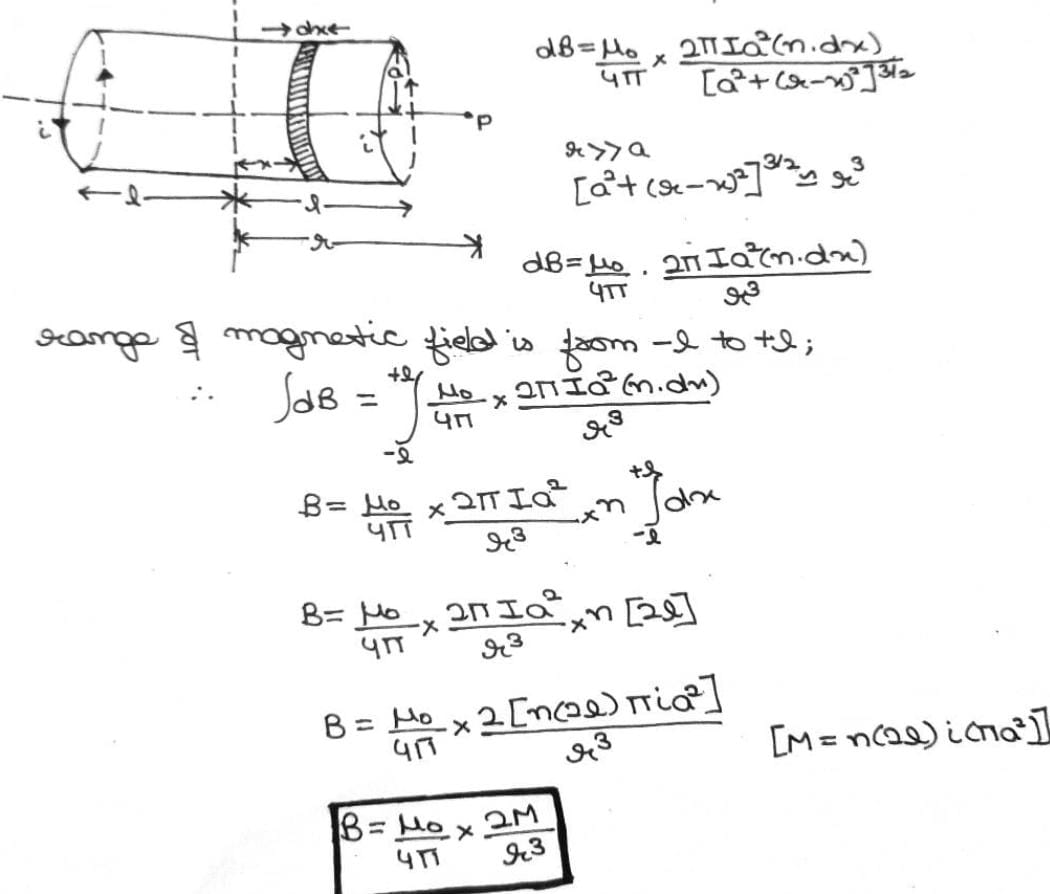Source

• The Electrostatic Analog

The analogy between electric and magnetic dipoles is summarized in the table below.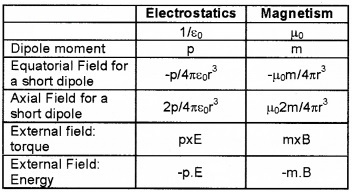Source

• Gauss’ Law and Magnetism

Through any closed surface, the net magnetic flux (B) is always zero.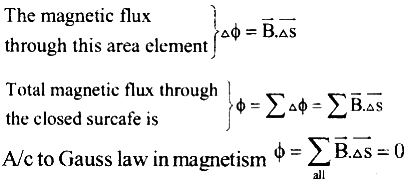Source

]]>